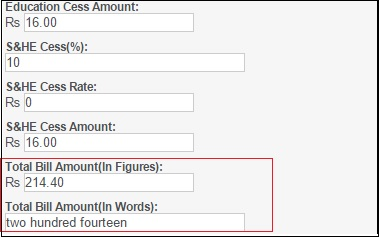# JavaScript to print Amount (In Words)

By | November 5, 2015

As you know, we have been writing blogs exploring the various features implemented in Sage CRM. With the help of JavaScript codes or say Client side API, customization efforts of the programmer’s life has come to an ease. We have already posted few blogs about writing JavaScript codes in .js files and calling the same in Custom Content of any screen.

Recently we came across a requirement, where we had to display an Amount summed up (In figures) into words. Thought of sharing this post, as it would help many of the Billing departments to print Billing Invoices from CRM.

New Stuff: Chat Rooms within Sage CRM

To achieve this functionality you can refer below written script. Simply, create a new js file and call the same on the field where you need the display the output.

<Snippet>
//declaring arrays and assigning values to them.
var th = [”,’thousand’, ‘million’, ‘billion’, ‘trillion’];
var dg = [‘zero’,’one’,’two’,’three’,’four’, ‘five’,’six’,’seven’,’eight’,’nine’];
var tn=[‘ten’,’eleven’,’twelve’,’thirteen’,’fourteen’,’fifteen’,’sixteen’,’seventeen’,’eighteen’,’nineteen’];
var tw = [‘twenty’,’thirty’,’forty’,’fifty’, ‘sixty’,’seventy’,’eighty’,’ninety’];
//function for converting numbers to words
Function toWords(s)
{
s = s.toString();
s = s.replace(/[\, ]/g,”);
if (s != parseFloat(s)) return ‘not a number’;
var x = s.indexOf(‘.’);
if (x == -1)
x = s.length;
if (x > 15)
return ‘too big’;
var n = s.split(”);
var str = ”;
var sk = 0;
for (var i=0; i < x; i++)
{
if ((x-i)%3==2)
{
if (n[i] == ‘1’)
{
str += tn[Number(n[i+1])] + ‘ ‘;
i++;
sk=1;
}
else if (n[i]!=0)
{
str += tw[n[i]-2] + ‘ ‘;
sk=1;
}
}
else if (n[i]!=0)
{
str += dg[n[i]] +’ ‘;
if ((x-i)%3==0) str += ‘hundred ‘;
sk=1;
}
if ((x-i)%3==1)
{
if (sk)
str += th[(x-i-1)/3] + ‘ ‘;
sk=0;
}
}
if (x != s.length)
{
var y = s.length;
str += ‘point ‘;
for (var i=x+1; i<y; i++)
str += dg[n[i]] +’ ‘;
}
return str.replace(/\s+/g,’ ‘);
}
</Snippet

On calling the script from the Custom Content section of CRM, output will be displayed as shown below.